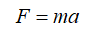# What would the slope of a line be of force was plotted on the x axis and acceleration was plotted on the y axis

Question
28 views

What would the slope of a line be of force was plotted on the x axis and acceleration was plotted on the y axis

check_circle

star
star
star
star
star
1 Rating
Step 1

Let F be the force, m be the mass of the particle, a be the acceleration, ms be the slope of the line.

Write the expression for Newton’s second law of motion....

### Want to see the full answer?

See Solution

#### Want to see this answer and more?

Solutions are written by subject experts who are available 24/7. Questions are typically answered within 1 hour.*

See Solution
*Response times may vary by subject and question.
Tagged in